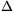Mathematical and Physical Journal
for High Schools
Issued by the MATFUND Foundation
 Already signed up? New to KöMaL?

#Problem P. 4460. (September 2012)

P. 4460. 31.4 J of heat is given to a sample of ideal gas. Because of this the gas expands from a volume of 0.3 litres to 0.8 litres, at a constant pressure of 1.57.104 Pa.

a) Determine the change in the internal energy.

b) What is the degree of freedom of the gas?

(4 pont)

Deadline expired on October 10, 2012.

Sorry, the solution is available only in Hungarian. Google translation

Megoldásvázlat. a)E=23,55 J;

b) f=6.

### Statistics:

 168 students sent a solution. 4 points: 145 students. 3 points: 4 students. 2 points: 9 students. 1 point: 5 students. 0 point: 5 students.

Problems in Physics of KöMaL, September 2012